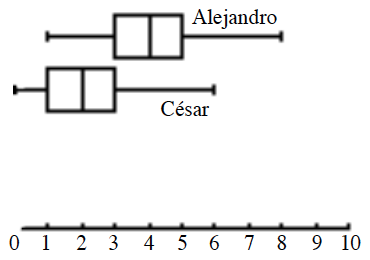### Home > ACC7 > Chapter 8 Unit 5 > Lesson CC2: 8.3.2 > Problem8-77

8-77.

Scientists studying prehistoric birds have estimated the weights (in grams) of two different sub-species of birds. The data they collected is shown below.   (Desmos)

Pre-emergent thrushes: $49$, $54$, $52$, $58$, $61$, $72$, $73$, $78$, $73$, $82$, $83$, $73$, $61$, $67$, $68$

Post-emergent thrushes: $65$, $35$, $48$, $29$, $57$, $87$, $94$, $68$, $86$, $73$, $58$, $74$, $85$, $91$, $88$, $97$

1. Create a parallel box plot to compare the weights of these two sub-species of birds.

Review the Math Notes box below from Lesson for help in making your plot.

Sometimes comparing only the two box plots is enough to be able to compare the distributions. When two box plots are drawn on the same axis, they are called parallel box plots. An example is shown at right.2. Would the mean accurately represent the middle of the data? Why or why not?

Remember that the mean is the average of the data. Is the average of this data in the middle?

3. A fossil of a bird is found. Scientists estimated that its weight must have been around $75$ grams. Which sub-species was the bird most likely a member of? Explain.

Post-emergent thrushes

Use the eTool below to complete the problem.
Click the link at right for the full version of the eTool: (Desmos)